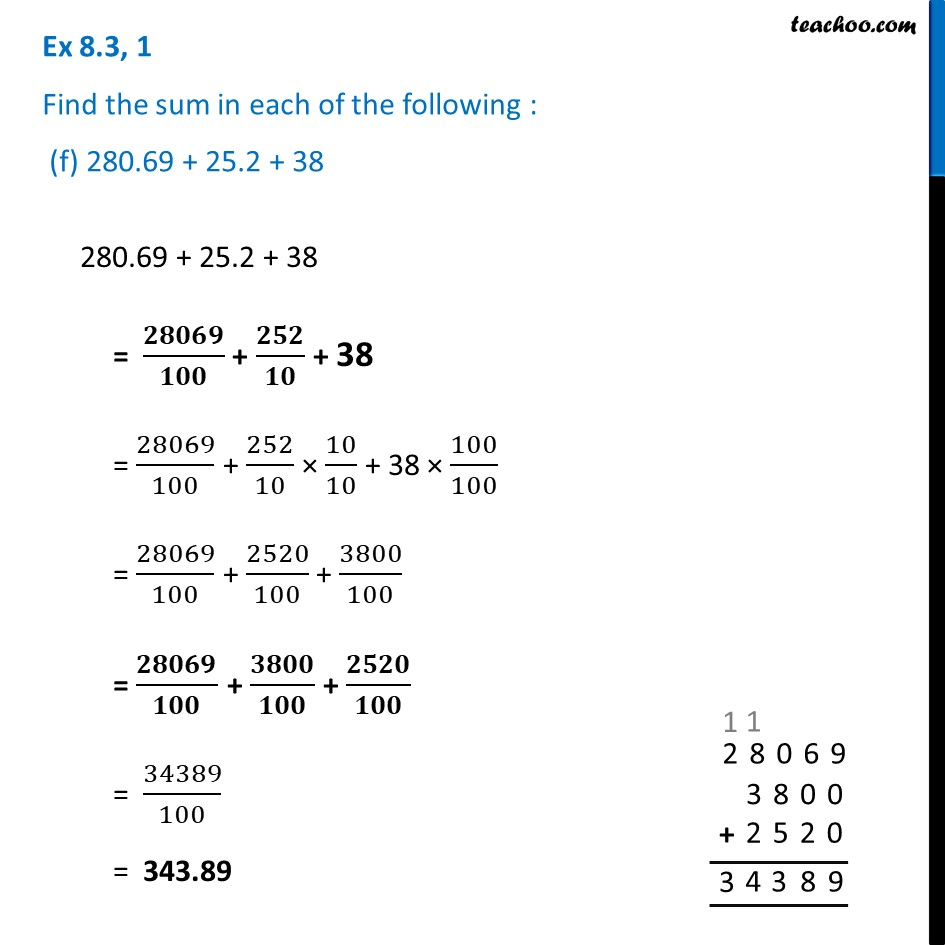Ex 8.3

Chapter 8 Class 6 Decimals
Serial order wiseLearn in your speed, with individual attention - Teachoo Maths 1-on-1 Class

### Transcript

Ex 8.3, 1 Find the sum in each of the following : (f) 280.69 + 25.2 + 38 280.69 + 25.2 + 38 = 𝟐𝟖𝟎𝟔𝟗/𝟏𝟎𝟎 + 𝟐𝟓𝟐/𝟏𝟎 + 38 = 28069/100 + 252/10 × 10/10 + 38 × 100/100 = 28069/100 + 2520/100 + 3800/100 = 𝟐𝟖𝟎𝟔𝟗/𝟏𝟎𝟎 + 𝟑𝟖𝟎𝟎/𝟏𝟎𝟎 + 𝟐𝟓𝟐𝟎/𝟏𝟎𝟎 = 34389/100 = 343.89